# ISEE Upper Level Math : How to find the least common multiple

## Example Questions

2 Next →

### Example Question #11 : How To Find The Least Common Multiple

What is the least common multiple ofand?Explanation:

Find all of the multiples of both numbers: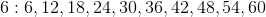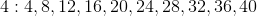Find the first number that both sets have in common.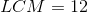### Example Question #12 : How To Find The Least Common Multiple

Find the LCM of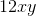and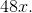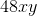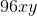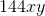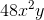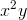Explanation:

LCM is the least common multiple of the pair. There are a couple of terms at play here, so first find the LCM of 12 and 48. Since 12 goes into 48, 48 is the LCM. Then, look at xy and x. XY is the LCM between those two. So, multiply those together to getas your answer.

### Example Question #13 : How To Find The Least Common Multiple

What is the least common multiple ofand?Explanation:

A multiple of x is a number that results when x is multiplied by another whole number.

The least common multiple of two numbers is the smallest number that is a multiple of both the numbers.

24 is a multiple of 8 because 8 times 3 is 24.

24 is also a multiple of 12 because 12 times 2 is 24.

There are no numbers that are smaller than 24 which are also multiples of 8 and 12. Therefore, 24 is the least common multiple.

2 Next →